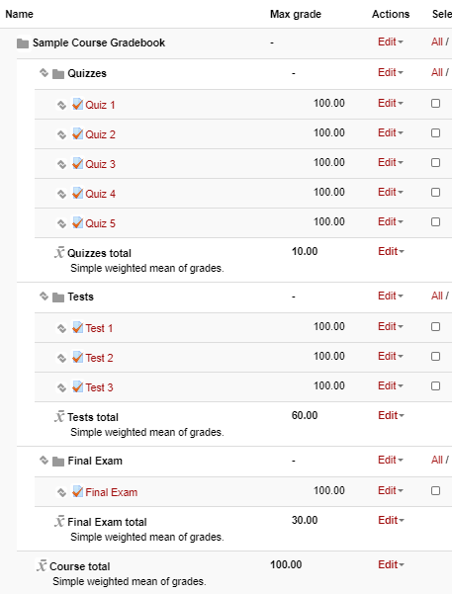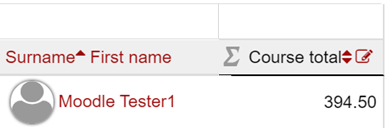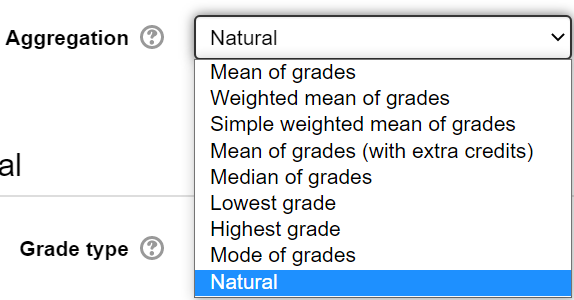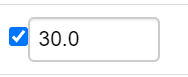# Grading Policy Based on Percentages

If a Syllabus Grading policy is based upon percentages, the aggregation method Simple Weighted Mean of Grades is effective. An example is a syllabus which states:

Quiz Average = 10%

Test Average = 60%

Final Exam = 30 %

The course contains 5 Quizzes worth 100 points each, 3 Tests worth 100 points each, and 1 Final Exam worth 100 points.

## The Gradebook Setup can be set up as follows:## What will the Instructor See?

The instructor will see each student's grade and a Course Total out of 100 percentage points.

## To replicate this:

1. From Gradebook Setup, locate the top-most folder icon. Click on Edit, then Edit Settings. Under Aggregation method, select Simple Weighted Mean of Grades. Then Save Changes.2. Still in Gradebook Setup, create new Categories for Quizzes, Tests, and the Final Exam. (Read more about Creating Categories in the Moodle Gradebook).

3. When creating these, set the Maximum Grade for the Category equal to the percentage value from the Syllabus. In our Example:

• The Maximum Grade for the Quizzes Category is 10 representing the 10% value from the Syllabus
• The Maximum Grade for the Tests Category is 60 representing the 60% value from the Syllabus
• The Maximum Grade for the Final Exam Category is 30 for the 30% value from the Syllabus.

4. Move currently existing grade items or create new grade items organized into the appropriate Category.

# Grading Policy Based on Total of Points

If a Syllabus Grading policy is based upon course points only, the Natural method is effective. An example is a syllabus which states:

5 Quizzes at 10 points each = 50 points

3 Tests at 100 points each = 300 points

1 Final Exam = 150 points

Course Total = 500 Points total

## The Gradebook Setup can be set up as follows:## What will the Instructor See?

The instructor will see each student's grade and a Course Total out of the total possible points (500 in this example)## To replicate this:

1. From Gradebook Setup, locate the top-most folder icon. Click on Edit, then Edit Settings. Under Aggregation method, select Natural. Then Save Changes.2. Confirm that the total number of points from the syllabus matches the Course Total (500 in this example).

3. Even though this example did not utilize them, Categories can also be created to fit specific gradebook needs.

4. If the weighting on any grade item needs to be changed, click on the checkbox in the Weights category. The weights of all other items will automatically respond to any changes made to the weighting.